# Quartz CrystalTheory

## AT-Cut Crystals

For precise frequency control in radio and line communication systems, quartz crystal resonators have proved indispensable. The material properties of crystalline quartz are such that quartz resonators display stabilities and Q factors that can not be matched by other types of resonator over a frequency range from a few kHz to 200 MHz.

## Frequency Temperature Characteristics

The AT-cut crystal has a frequency temperature characteristic which may be described by a cubic function of temperature.

This characteristic can be precisely controlled by small variations in the exact angle at which the crystal blank is cut from the original quartz bar. Fig. 1 illustrates some typical cases. This cubic behaviour is in contrast to most other crystal units, which have parabolic temperature characteristics.

As a consequence, the AT-cut is generally the best choice when specifying a unit to operate over a wide temperature range, and is available in a range of frequencies from 1 to 200 MHz.## Equivalent Circuit

Fig. 2 shows the conventionally accepted equivalent circuit of a crystal resonator at a frequency near its main mode of vibration. The inductance L1 represents the vibrating mass, the series capacitance C1 the compliance of the quartz element, and the resonance R1 the internal friction of the element, mechanical losses in the mounting system, and acoustical losses to the surrounding atmosphere.

The shunt capacitance CO is made up of the static capacitance between the electrodes, together with stray capacitance of the mounting system.

L1 = Dynamic or Motional Inductance (mH)
C1 = Dynamic or Motional Capacitance (fF) (10-15F)
R1 = Equivalent Series Resistance (ohms)
CO = Parallel or Static Capacitance (pF)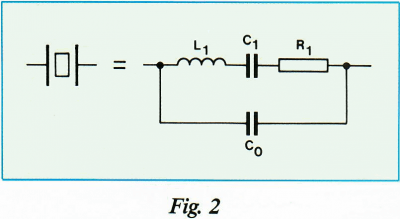There are two zero-phase frequencies associated with this simple circuit; one is at series resonance fs, another at anti-resonance fa. When used in an oscillator at any frequency within the broken lines of Fig. 3 as determined by the phase of the maintaining circuit.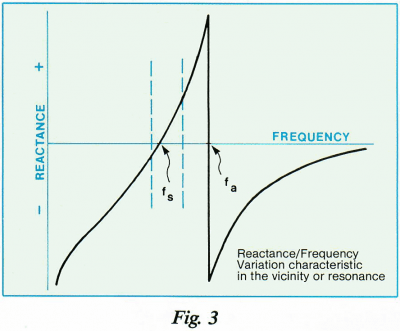By variation of this reactive condition the crystal frequency may be trimmed to a limited extent. The degree to which this frequency may be varied (frequency pulling) is inversely proportional to the capacitance ratio.## Load Capacitance & Frequency Pulling

Many practical oscillator circuits make use of a load capacitor CL in series or parallel with the crystal, either in order to provide a means for final frequency adjustment, or perhaps for modulation or temperature compensation purposes. The presence of the load capacitor shifts the operating frequency of the crystal by an amount dependent on the value of CL and the values of C0 and C1. The fractional difference in frequency between the load resonance  frequency FL and the series resonance frequency FS is known as the load resonance frequency offset (L.O.).In many applications a variable capacitor (trimmer) is used as the load reactive element to adjust the frequency. The fractional frequency range available between specified values of the load reactive element is called the pulling range (P.R.) and it can be calculated by using the following formula:A useful parameter to the design engineer is the pulling sensitivity (S) at a specified value of load capacitance. It is defined as the incremental fractional frequency change for an incremental change in load capacitance. It is normally expressed in ppm/pf (106/pf) and can be calculated from the formula:It is very important to define the mean load capacitance to enable the actual crystal frequency to fall within the tolerances of the specified nominal frequency. It is also important to use, wherever possible, standard values of load capacitance; for example 20pf, 30pf.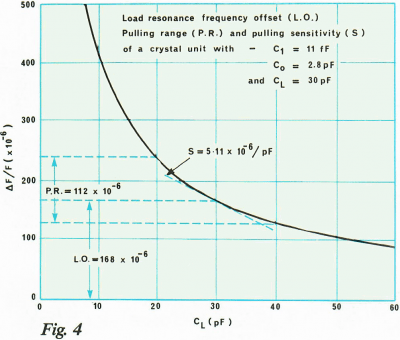Fig. 4 shows the relationship between L.O., P.R. and S.

The equivalent circuit of the crystal has one other important parameter: This is R1, the motional resistance. This parameter controls the Q of the crystal unit and will define the level of oscillation in any maintaining circuit. The load resonance  resistance for a given crystal unit depends upon the load capacitance with which that unit is intended to operate. The frequency of oscillation is the same in either a series or parallel connection of the load capacitance. If the external capacitance is designated, the load resonance resistance may be calculated as follows: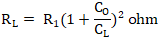The equivalent shunt or parallel resistance at the load resonance frequency is approximately:It should be remembered that R1 does not change, thus the effective parameters of any user network can be readily calculated.

## Frequency Pulling Calculation

An approximation to the pulling of any crystal can be calculated from this simple formula:## Circuit Equations - Basic Crystals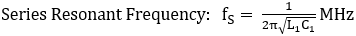Note: In practice, an allowance usually has been made for ‘strays’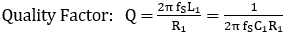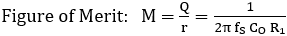## Circuit Equations - with external load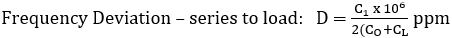Note 1: Series Capacitance (CL) increases with frequency.

Note 2: The effect of a trimmer may be readily assessed by calculating D & D’ for CL & CL and subtracting.

Note 3: Units are correct for C1, CO and CL in Farads.Fig. 5 showing the equivalent circuit with an external load (CL)Fig. 6

Fig. 6 shows the effect of external load capacitance on the trim sensitivity of a representative 20MHz fundamental mode crystal. Notice the large frequency shift with small load changes when fundamental mode crystals are operated at low load capacitance’s. Overtone mode crystals are generally less affected by external loads. A rough approximation is to reduce the fundamental mode trim sensitivity by a factor of the overtone mode crystal.# Find at Amazon and other good book sellers## Thank You

for getting in touch with us.
We’ll be contacting you soon.## Thank You

for submitting your request for quotation.
We’ll be in contact soon.